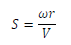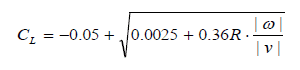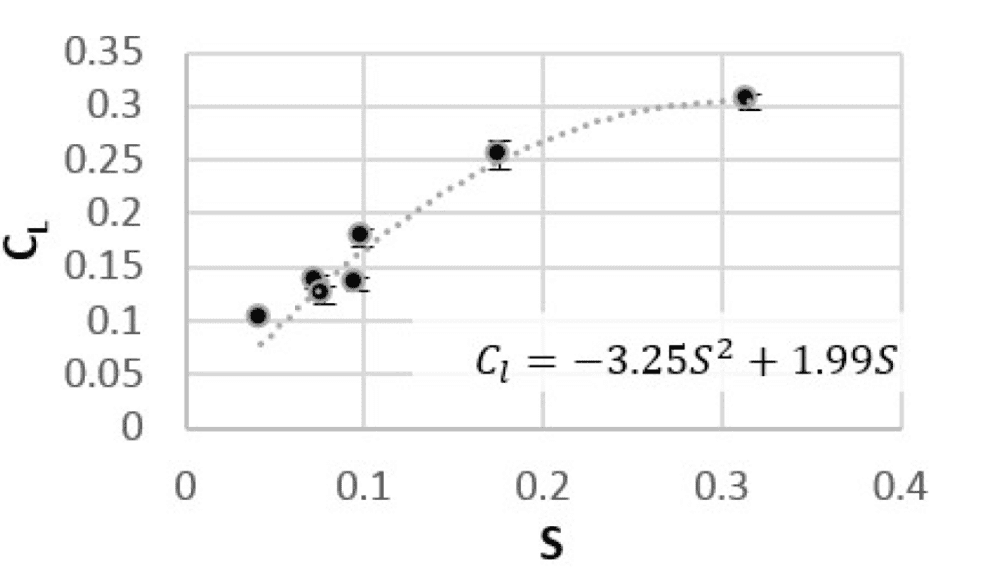# What units are used in spin factor and coefficient of lift formulas?

FGD
I am trying to add the magnus effect to a golf simulator program but am getting stuck on the coefficient of lift. What are the units I should be using in these calculations? The rest of my program I use SI units. But I seam to get weird results when using them with these equations.

Spin factor = Angular Velocity * radius / velocityCoefficient of lift = -0.05 + sqrt(0.0025 + 0.36 * radius * abs[angular velocity/velocity])I've seen angular velocity in rpm or rad/s or...
I've seen velocity in mph or m/s or...
I've seen radius in inches or meters or...

The Coefficient of lift formula is taken from this paper.
The Spin factor from this paper.

If I get the proper units it should provide a graph with similar results to these. Left coefficient of lift, bottom spin factor.If you know of better research papers or source code, I would love a link.
An example problem would also be very helpful.

Thanks

What are the units I should be using in these calculations?
The spin factor looks dimensionless to me.

FGD
Yeah, both are supposed to be dimensionless. I was getting all kinds of weird results last night. (Maybe too tired) I tried again with SI units and calculations work now...